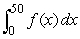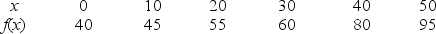# [Solved] Use the Table to Estimate with N = 5

Question 19
Essay

## Use the table to estimatewith n = 5 and x = 10.(Average left-and right-hand sums).10+ million students use Quizplus to study and prepare for their homework, quizzes and exams through 20m+ questions in 300k quizzes.

### Chemistry

Explore our library and get Introductory / Preparatory Chemistry Homework Help with various study sets and a huge amount of quizzes and questions

145

Study sets

3.2K

Quizzes

275.8K

Questions

Upload material to get free accessInvite a friend and get free accessSubscribe and get an instant access